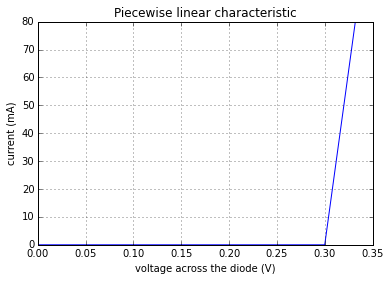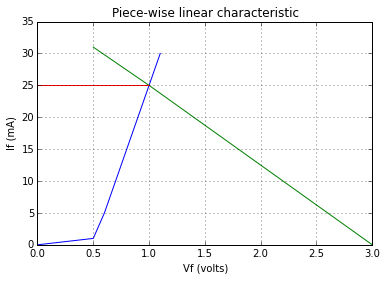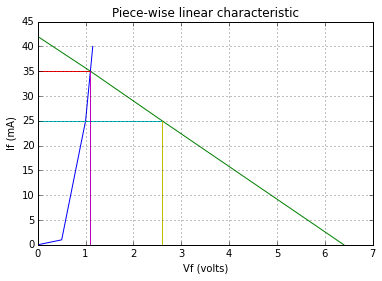# Chapter 2 Semiconductor devices¶

## Ex 2.1 Pg-2.18¶

In :
Vf=0.2 #  #voltage in volts
Vr=60 #  #voltage in volts
If=60*10**(-3) #  #current in  ampere
I0=0.025*10**(-3) #  #current in  ampere
Rf=Vf/If # #forward resistance
Rr=Vr/I0 # #reverse resistance
Rr=Rr*1e-6
print "the equivalent resistance are Rf=%.3f ohm and Rr=%-.1f M ohm"%(Rf,Rr)

the equivalent resistance are Rf=3.333 ohm and Rr=2.4 M ohm


## Ex-2.2 Pg-2.18¶

In :
print "refer to the Figure-2.19 given "
print "from the characteristics at point P, Vf=0.7V,If=60mA"
Vf=0.7 #
If=0.06 #
Rf=Vf/If # #DC forward resistance
print "\n DC forward resistance Rf : %.2f ohm\n"%(Rf)
print "as the forward voltage changes from P to Q"
delta_Vf=0.77-.7 #
delta_If=(120-60)*10**(-3) #
rf=delta_Vf/delta_If # #dynamic forward resistance
print "\n Dynamic forward resistance rf : %.3f ohm"%(rf)

refer to the Figure-2.19 given
from the characteristics at point P, Vf=0.7V,If=60mA

DC forward resistance Rf : 11.67 ohm

as the forward voltage changes from P to Q

Dynamic forward resistance rf : 1.167 ohm


## Ex 2_3 Pg-2-22¶

In :
print " Refer to the figure-2.24 shown "
print " since Rf=0 The circuit becomes as shown in figure-2.24(a)"
V=10 # #supply voltage
Rf=0 # #forward resistance
Rl=1 # #load resistance in k ohm
Vin=0.7 # #cut in voltage
Il=(V-Vin)/Rl # #applying KVL to the loop
If=Il #
print "\n \n current through the resistance Il=If = is %.1f mA"%(If)
Vl=Il*Rl #
print "\n \n voltage across Rl is %.1f V"%(Vl)
Pd=If*Vin #
print "\n \n diode power Pd = %.2f mW"%(Pd)
Pl=Il*Vl #
print "\n \n load power Pl = %.2f mW"%(Pl)

 Refer to the figure-2.24 shown
since Rf=0 The circuit becomes as shown in figure-2.24(a)

current through the resistance Il=If = is 9.3 mA

voltage across Rl is 9.3 V

diode power Pd = 6.51 mW

load power Pl = 86.49 mW


## Ex 2_4 PG-2.23¶

In :
print "Refer the Figure-2.25 shown"
print "When forward resistance Rf is neglected  then"
print "the diode behaves as a battery as shown in Figure-2.25(a)"
Vf=0.7 # #cut-in voltage
V=10 # #supply voltage
If=(V-Vf)/Rl # #applying KVL to the circuit
If=If*1e3
print "\n Forward current is %.2f mA\n"%(If)
print "When forward resistance is Rf is 3.2 Ohm then"
print "the equivalent circuit is as shown in fig-2.25(b)"
Rf=3.2 #
If=(V-Vf)/(Rl+Rf) # #applying KVL to the circuit
If=If*1e3
print "\n therefore Forward current is %.4f mA"%(If)

Refer the Figure-2.25 shown
When forward resistance Rf is neglected  then
the diode behaves as a battery as shown in Figure-2.25(a)

Forward current is 18.60 mA

When forward resistance is Rf is 3.2 Ohm then
the equivalent circuit is as shown in fig-2.25(b)

therefore Forward current is 18.4817 mA


## Ex 2_5 PG-2.23¶

In :
%matplotlib inline
import matplotlib.pyplot as plt

If=80e-3 # #maximum forward current
Rf=0.4 # #dynamic resistance
Vin=0.3 # #cut-in voltage for germanium
print "when forward current is zero then"
Vf=Vin # #voltage across the diode
print " voltage across the diode is %1.1f V\n"%(Vf)
print "when forward current is 80mA then"
Vf=Vin+If*Rf #
print " voltage across the diode is %1.3f V"%(Vf)
x=[0 ,.1 ,.2 ,.3 ,.332] # #x-coordinate
y=[0  ,0  ,0  ,0  ,80] # #y-coordinate
plt.plot(x,y)
plt.xlabel('voltage across the diode (V) ') #
plt.ylabel('current (mA)') #
plt.title('Piecewise linear characteristic')
plt.grid()
plt.show()

when forward current is zero then
voltage across the diode is 0.3 V

when forward current is 80mA then
voltage across the diode is 0.332 V## Ex 2_6 PG-2.28¶

In :
%matplotlib inline
import matplotlib.pyplot as plt

print "Refer to the Figure:2.29 shown"
If=25e-3 # #current at Q-point
print "for Q point If=25mA, ie Iq=25mA"
print "for If=0 A, Vf=Vin=3V at point B"
print "Now we draw the graph"
print "The coordinates are Q=(1V,25mA) B=(3V,0mA)"
x=[ 0 ,0.5, 0.6,    1,   1.1 ] # #x-coordinate
y=[ 0 , 1 ,  5     ,25   ,30 ] # #y-coordinate
plt.plot(x,y)
x1=[0.5 ,  1,    3] # #x-coordinate
y1=[ 31   ,25 ,  0] # #y-coordinate
plt.plot(x1,y1)
x2=[ 0, 1] #
y2=[25 ,25] #
plt.plot(x2,y2)
plt.xlabel('Vf (volts)') #
plt.ylabel('If (mA)') #
plt.title("Piece-wise linear characteristic")
plt.grid() #
plt.show()
print "Q-point is denoted by the intersection of two lines as shown in the plot"
delta_If=10e-3 # #from the graph plotted
delta_Vf=0.9 # #from the graph plotted
s=delta_If/delta_Vf # #slope
print "Therefore load resistance is the reciprocal of the slope "
print "\n required load resistance is %.0f ohm"%(Rl)

Refer to the Figure:2.29 shown
for Q point If=25mA, ie Iq=25mA
for If=0 A, Vf=Vin=3V at point B
Now we draw the graph
The coordinates are Q=(1V,25mA) B=(3V,0mA)Q-point is denoted by the intersection of two lines as shown in the plot
Therefore load resistance is the reciprocal of the slope

required load resistance is 90 ohm


## Ex 2_7 PG-2.29¶

In :
import matplotlib.pyplot as plt
%matplotlib inline

If=35e-3 # #current at which Q-point appears
print "We draw the new DC load line in the plot"
s=-1/Rl # #slope
print "\n slope is %f \n"%(s)
x=[ 0 ,0.5,  1,   1.1, 1.15 ] # #x-coordinate
y=[ 0  ,1 , 25   ,35  , 40 ] # #y-coordinate
plt.plot(x,y)
x1=[0, 1.1, 2.6, 6.4 ] # #x-coordinate
y1=[42  ,35  , 25 , 0] # #y-coordinate
plt.plot(x1,y1)
x2=[ 0 , 1 , 1.1] # #x-coordinate
y2=[35 ,35 , 35] # #y-coordinate
plt.plot(x2,y2)
x3=[0 , 1 , 2.6] # #x-coordinate
y3=[25 ,25 ,25] # #y-coordinate
plt.plot(x3,y3)
x4=[1.1 ,1.1, 1.1] #
y4=[ 0  ,10  , 35 ] #
plt.plot(x4,y4)
x5=[2.6, 2.6] #
y5=[0  ,  25] #
plt.plot(x5,y5)
plt.xlabel('Vf (volts)') #
plt.ylabel('If (mA)') #
plt.title("Piece-wise linear characteristic")
plt.grid()
print "The slope passes through the Q-point so the equation of the load "
print "line is If=(-Vf/Rl)+(Vin/Rl)"
print "Now from the graph we can see that slope =(change in If)/(change in Vf)"
print "For If=35 mA Vf=1.1 V"
print "The coordinates are Q=(1.1V,35mA) C=(2.6V,25mA) B=(6.4V,0mA)"
delta_If=10e-3 # #change in If
delta_Vf=delta_If/abs(s) # #change in Vf and we take only the magnitudeof the slope
print "\n change in Vf is %1.1f V \n"%(delta_Vf)
print "This is point C corresponding to If=35-10=35mA, as If is change by 10mA"
print "and Vf=2.6V "
print "We join the Q-point and point C as shown in the plot to get the DC load"
print " line we extend the line to intersect point B "
print "the voltage at point B is the new supply voltage required"
print "\n From the plot voltage at point B = 6.7 V"

We draw the new DC load line in the plot

slope is -1.000000

The slope passes through the Q-point so the equation of the load
line is If=(-Vf/Rl)+(Vin/Rl)
Now from the graph we can see that slope =(change in If)/(change in Vf)
For If=35 mA Vf=1.1 V
The coordinates are Q=(1.1V,35mA) C=(2.6V,25mA) B=(6.4V,0mA)

change in Vf is 0.0 V

This is point C corresponding to If=35-10=35mA, as If is change by 10mA
and Vf=2.6V
We join the Q-point and point C as shown in the plot to get the DC load
line we extend the line to intersect point B
the voltage at point B is the new supply voltage required

From the plot voltage at point B = 6.7 V## Ex 2_8 PG-2.33¶

In :
from math import exp
V=0.22 # #forward bias voltage
T=25+273 # #room temperature in degree kelvin
I0=2e-3 # #reverse saturation current
n=1# #for germanium diode
k=8.62e-5 #Boltzmann's constant
Vt=k*T #
I=I0*(exp(V/(n*Vt))) # # diode current
print "therefore the P-N junction diode current is %f A"%(I)
# in the book they have taken the approximate value
#hence the answer is slighty different. in the book
#Vt=0.02568(approx) whereas Vt=0.0256876(exact value)

therefore the P-N junction diode current is 10.483844 A


## Ex 2_9 PG-2.36¶

In :
P1=500e-3 # #rated power rating of the diode
T1=27 # #temperature in Degree Celsius
Df=4e-3 # #Derating factor
print "At temperature T=27 degree C"
print "For silicon diode Vf=0.7 V is constant "
Vf=0.7 #
If1=P1/Vf #
print " \n Therefore maximum forward current at T=27 degree C is %.4f A \n"%(If1)
print "At temperature T=77 degree C"
T2=77 # #temperature in degree celsius
P2=P1-(T2-T1)*Df # #rated power rating of the diode at T=77 degree C
If2=P2/Vf #
print "\n Therefore maximum forward current at T=77 degree C is %.4f A"%(If2)

At temperature T=27 degree C
For silicon diode Vf=0.7 V is constant

Therefore maximum forward current at T=27 degree C is 0.7143 A

At temperature T=77 degree C

Therefore maximum forward current at T=77 degree C is 0.4286 A


## Ex 2_10 PG-2.37¶

In :
T1=27 # #initial temperature
Vfl=0.7 # #forward voltage
Vtc=-2.3e-3 # #voltage temperature coefficient
print "at T2=25 degree C"
T2=50 #
Vf2=Vfl+((T2-T1)*Vtc)
print "\n therefore forward voltage drop at 50 degree C is %.4f V \n"%(Vf2)
print "at T3=77 degree C"
T3=77 #
Vf2=Vfl+((T3-T1)*Vtc)
print "\n therefore forward voltage drop at 77 degree C is %.3f V"%(Vf2)

at T2=25 degree C

therefore forward voltage drop at 50 degree C is 0.6471 V

at T3=77 degree C

therefore forward voltage drop at 77 degree C is 0.585 V


## Ex 2_11 PG-2.38¶

In :
If=30e-3 # #forward current
T1=25+273 # #temperature in degree kelvin
print "Therefore at a temperature of 25 Degree C "
Rf=26e-3/If #
print "\n dynamic resistance is %.3f ohm \n"%(Rf)
print "Therefore at a temperature of 75 Degree C "
T2=75+273 #Temperature in degree kelvin
Rf=Rf*(T2/T1) #
print "\n dynamic resistance is %.3f ohm"%(Rf)

Therefore at a temperature of 25 Degree C

dynamic resistance is 0.867 ohm

Therefore at a temperature of 75 Degree C

dynamic resistance is 0.867 ohm


## Ex 2_12 PG-2.43¶

In :
Tf_min=1 # #fall time in micro second
Trr_max=Tf_min/10
print "the maximum recovery time is %.1f micro sec"%(Trr_max)

the maximum recovery time is 0.0 micro sec


## Ex 2_13 PG-2.48¶

In :
PD_max=320e-3 # #maximum power rating
T1=50 # #temperature in degree celsius at which maximum power rating occurs
DF=2.3e-3 # #Derating factor
print "Therefore the derated power rating at a temperature T=100 Degree celsius"
T2=100 #
PD=PD_max-DF*(T2-T1) #
print "\n is %.3f W"%(PD)

Therefore the derated power rating at a temperature T=100 Degree celsius

is 0.205 W


## Ex 2_14 PG-2.52¶

In :
Vzd=50e-3 # #change in Vz
Izd=2.5e-3 #change in Iz
Zz=Vzd/Izd # #zener resistance
print "zener resistance Zz=%.0f ohm"%(Zz)

zener resistance Zz=20 ohm


## Ex 2_15 PG-2.52¶

In :
rz=4 # #zener diode resistance
Vz=5.1 #
Iz=25e-3 #
x=Iz*rz #
Vzz=Vz+x # #total terminal voltage across the diode
print "Therefore total terminal voltage across the diode Vz''= %.1fV"%(Vzz)

Therefore total terminal voltage across the diode Vz''= 5.2V


## Ex 2_16 PG-2.52¶

In :
Vz=6.8 #nominal voltage
Pd_max=500 # #zener diode power loss in mW at 40 degree celsius
D=2.6 # #Derating factor in mW/degree celsius
T1=40 # #Temperature in degree celsius
Izm=Pd_max/Vz #
print "   At T=40 degree celsius maximum zener current Izm=%.2f mA\n\n"%(Izm)
T2=100 # #Temperature in degree celsius
delta_T=T2-T1 # #change in temperature
Pd_derated=Pd_max-D*delta_T
Izm=Pd_derated/Vz #
print "   At T=100 degree celsius maximum zener current Izm=%.3f mA\n\n"%(Izm)

   At T=40 degree celsius maximum zener current Izm=73.53 mA

At T=100 degree celsius maximum zener current Izm=50.588 mA



## Ex 2_17 PG-2.52¶

In :
print "Refer to the figure-2.51 shown\n"
print " We apply KVL across the circuit \n"
print " Therefore -Iz*R1-Vz+Vin = 0 \n"
Vin=15 #
Vz=5.2 #
R1=670 #
Iz=(Vin-Vz)/R1 # #zener diode current
Iz=Iz*1e3 # #in mA
Pd=Vz*Iz # #power dissipation
print " \n zener diode current Iz:%f mA\n power dissipation :%f mW "%(Iz,Pd)

Refer to the figure-2.51 shown

We apply KVL across the circuit

Therefore -Iz*R1-Vz+Vin = 0

zener diode current Iz:14.626866 mA
power dissipation :76.059701 mW